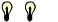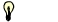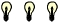# The Python Visual Sandbox

visualizing the invisible   -   explicating the implicit

Michael Weigend 17. 7. 2004 - 21.12. 2005Check your activities]   [register]   [gallery]   [coaching]   [workshops]   [highscores]   [usage]

#### What is the Python Visual Sandbox?

The Python Visual Sandbox is a collection of interactive online applications containing animations which visualize the execution of Python programs.  You can experiment and practise programming "in micro-scale" in order to get a better understanding of algorithms and Python programming technique. The tasks differ in their level of difficulty. Note the light bulbs!

#### Registration

You need a password for playing in the Python Visual Sandbox. Please register to obtain a password. It takes only a minute.

#### Points

Playing in the Python Visual sandbox you can earn credits (points). For each Python Visual (only first session) you get 20 points.  You just have to watch each animation from the beginning to the end at least one time and answer the questions at the end. In Python Puzzle and Python Quiz it depends on your performance, how many points you earn. If you want to know how many points you have collected yet, click here.

#### Python Visual

Watch movies that visualize a small Python program or some programming principle in different ways. Decide, which animation explains best!regular expressions Regular expressions are patterns which match certain strings. Imagine, how they work.list Lists are mutable objects. What does this mean?iteration Judge different analogies for iterations.multilist Lists are mutable objects. Discover the magic of changes in a list of lists (multilist).factorial How does a recursive function compute the factorial  n! ?Fibonacci How does a recursive function compute the n-th Fibonacci number?mirror A recursive function arranges the letters of a word in reverse order.  It turns "roma" to "amor". How does this work?sort A list of numbers is sorted applying the algorithm "straight selection"return What happens, when a function returns something?

#### Python Puzzle

In a Python Puzzle you can manipulate fragments of program code on the screen. Build a program using given statements and test it! If your program is a logically correct solution of the problem, you earn points. A Python Puzzle is somewhat like a riddle. There are animations which help you finding the solution.recursion Define a function that counts the occurencies of a certain letter in a string.straight selection Define functions that sort a list of numbers.multilist We model a group of persons using a list of lists. Define functions that access this list. There are 3 tasks and one hint for each task which illustrates how the function works.  Maximal time is 600 seconds. Be quick in order to get bonus pointsmultilist (part 2) We model a group of persons using a list of lists. Define more functions which access this list. There are 4 tasks  and one hint for each task.  Maximal time is 800 seconds.

The following puzzles are about assertions. An assertion is a logical condition that must be true while the program is running. Using assert statements you can verify the logical correctness of your program. When  an assertion fails, the interpreter stops the execution of the program and raises an exception (assertion error). This helps the programmer to find the logical error in the script.first steps Practise the use of assert statements and make a Python script "logically safe". The more (correct) assertions you can pu into the script the more points you earn.reverse words There are three programs which arrange the letters of a word in reverse order. Add as many assertions as possible!assertions for quicksort Quicksort is a very fast sorting algorithm invented by Hoare. Increase the "logical safety" of different Quicksort implementations by adding assert statements. This is also a nice exercise in logic.

#### Python Quiz

How well can analogies illustrate a running program? In a Python Quiz you have to decide whether an analogy fits to a Python statement or not.  When you are quick, you get bonus points. But be careful! Wrong assignments will lead to penality points!assign You see very simple Python programs just consisting of a few assign statements.  Which visual models are appropriate visualizations of what is going on?list A group of persons is modeled by a list of tuples. A Python function takes such a list as input and returns the names of all persons that  are older than age. Which analogies fit to the statements of the program?objects In an object oriented program objects interact by exchanging messages. What does that mean?

#### Feedback

Any ideas, comments, criticisms or questions? Write an e-mail to me!
 (c) 2004- 2005  Michael Weigend